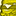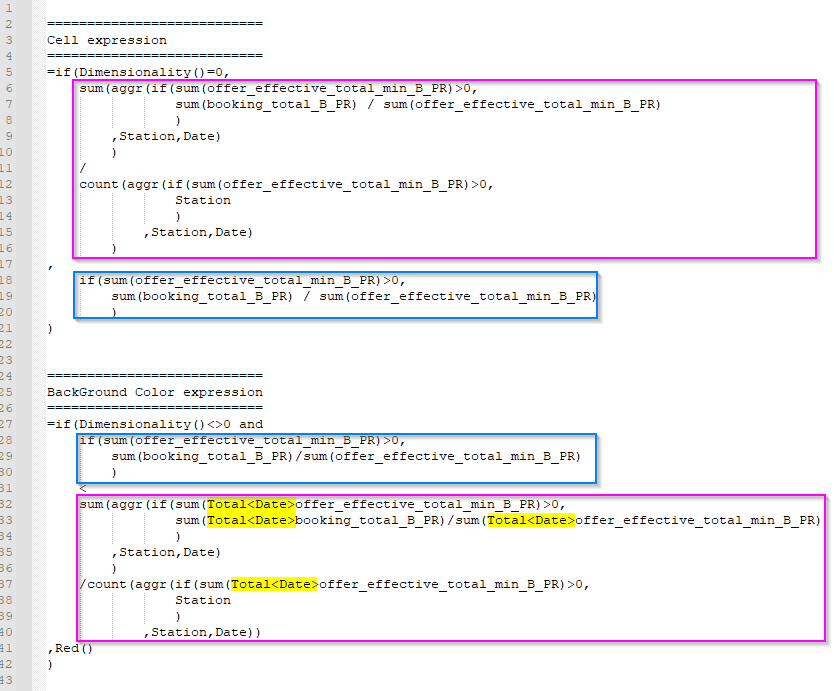# QlikView App Dev

Discussion Board for collaboration related to QlikView App Development.

cancel
Showing results for
Did you mean:Contributor II

## Changing background color of cell in pivot table depending on the total value of the column

Hello,

I have a pivot table with % of filling per day per center.

I'm trying to change the background color for the cells that are under the Total %.

The total is not the same expression as the rest of the cell.

My table as 2 dimensions:

-Station

-Date

My expression:

``````=if(Dimensionality()=0,
sum(aggr(if(sum(offer_effective_total_min_B_PR)>0,
sum(booking_total_B_PR)
/sum(offer_effective_total_min_B_PR)),Station,Date))
/count(aggr(if(sum(offer_effective_total_min_B_PR)>0,Station
),Station,Date))
,if(sum(offer_effective_total_min_B_PR)>0,
sum(booking_total_B_PR)
/sum(offer_effective_total_min_B_PR))
)``````

As you can see, my Dimensionality 0 is different then the rest.

Here is my expression for the background color:

``````=
if(Dimensionality()<>0 and if(sum(offer_effective_total_min_B_PR)>0,
sum(booking_total_B_PR)
/sum(offer_effective_total_min_B_PR))
<
sum(aggr(if(sum(Total<Date>offer_effective_total_min_B_PR)>0,
sum(Total<Date>booking_total_B_PR)
/sum(Total<Date>offer_effective_total_min_B_PR)),Station,Date))
/count(aggr(if(sum(Total<Date>offer_effective_total_min_B_PR)>0,Station
),Station,Date))
,Red()
)``````

Here is the result:As you can see this is close to what I want but I still have some calculation issue in the background expression. If you look at 2021-03-17 column you can see that the total is 85% yet some of the cells at 83% are not in red.

I can't figure out my error so if someone could point it to me that'd be great.

Thank you

Labels (1)
• ### Application Development

3 RepliesContributor III

Hi HugoR,
I would expect the Background color expression to reuse the same components as your Cell expression.

In the Background color expression, your components expressions have a "TOTAL<Date>" that is not present in the Cell expression. Is that on purpose? (See yellow)

If not, try to align your expressions (in purple frame)Contributor II
Author

Hi Denisg,

I had the same though as you and that's the first thing I tried but this doesn't work. None of the cells are red when using the same purple expression.

The Total<Date> is there on purpose. The cells are calculated using the date columns and the Station rows but I want to compare that value to a value that is calculated on the date column only. The key word TOTAL would allow me to apply my expression on all the data of my table. Adding <Date> will apply the expression on all my data for each date.

I'm not sure I explain it right.Contributor III

Hi,

I suppose you also tried to put the "Total<Date> in the blue frame of the Background expression ?
Are you able to send a sample of your data or qvw?